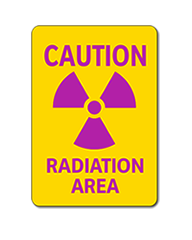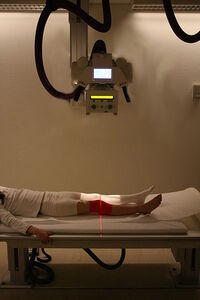# Monthly Archives: March 2015

## What is the Sum of Fractions Rule?

Dear Dr. Zoomie – I was reading about posting areas that hold radioactive materials and they said something about the “sum of fractions” that I didn’t quite understand. There was some mathematical equation, but I didn’t exactly understand that either. Can you tell me what’s up with the “sum of fractions?” Thanks!

This one is actually sort of important, but it’s not as hard as the regs make it seem. And you can use the same thinking for a couple of things, so it’s worth trying to understand. Here’s how it works.

Say you’ve got a room where you’re storing radioactive materials and you’re trying to figure out whether or not you need to put up a sign indicating it’s being used for radioactive materials storage. If you look in the regulations you’ll find a table telling you what level of activity requires licensing (anything less than this limit is exempt from licensing) and possibly another table telling you what level requires labeling (if you can’t find the second table then the labeling level is 10 times as high as the exemption limit). So it seems pretty simple – if you have more than the limit for labeling then you have to post the room and if you have less than the limit, you don’t. In reality it’s a little more complicated.

Let’s try an easy one – according to 10 CFR 30 Appendix B (which is titled Quantities of Licensed Materials Requiring Labeling) you will find that if you are storing tritium (H-3) in a room then you have to post the room if you have more than 1000 µCi (or 1 mCi). So if you have, say, 900 µCi of tritium in the room you don’t have to worry about posting it. Similarly, the level for P-32 is 10 µCi and the limit for I-125 (both of these are used in research) is 1 µCi. So if you have less than 10 µCi of P-32 or less than 1 µCi of I-125 you’re also exempt from having to post the room for radioactive materials storage. But what if you’ve got a bit of all of these nuclides – or others?

Say, for example, you have a room with 400 µCi of H-3, 4 µCi of P-32, and 0.4 µCi of I-125? None of these are high enough to require posting in and of themselves. But using the “sum of fractions” you need to put a sign on the room anyhow. The table here explains why.

 Nuclide Labeling limit (µCi) Amount on-hand (µCi) Fraction of limit H-3 1000 400 0.4 P-32 10 4 0.4 I-125 1 0.4 0.4 Sum of fractions 1.2

So – the first thing you need to do is to figure out how much activity you’ve got compared to the labeling limit (half, a third, three quarters, etc.). To do this you divide the amount of activity you’ve got by the labeling limit (for example, 400 µCi is 40% of the limit of 1000 µCi for tritium). After you’ve done this for each of the nuclides you’re storing you just add up all of the fractions – if the sum is greater than 1 you have to post the room.

So let’s take the table above. For each of these nuclides you have 40% of the allowable limit (40% is the same as 0.4). Since you have three nuclides, each of them with 40% of the allowable limit, the sum of the fractions comes out to 0.4+0.4+0.4+1.2. Since this is greater than 1.0 the room has to be posted. Easy, right?

You see the Sum of Fractions show up in a number of places. For example, 10 CFR 37 talks about when you have to take increased controls over the security of radioactive materials – and you use the sum of fractions if you have multiple nuclides in storage. If you’re discharging radionuclides into the sanitary sewer system (or letting them escape into the air) you’ll have limits for each radionuclide being discharged – the sum of fractions is used here as well. There’s more, but you get the idea. Once you understand how the idea works then you can use the same technique – the same calculations (or the same spreadsheet) across the board.

## Rad Area Posting – Millirem Per Hour Versus Millirem In An Hour (mR/hr v. mR in an hour)

Dear Dr. Zoomie – I work in a laboratory where we use x-ray machines on a regular basis. When the machine is on we have measured dose rates of up to 100 mR/hr in parts of the lab and as high as 50 mR/hr in the storage room on the other side of the wall from the x-ray machine. The previous RSO never posted these rooms as radiation areas, even though the regulations say that anyplace where dose rates are higher than 5 mR/hr have to be posted. Did my predecessor screw up, or do we have to post these rooms as radiation areas?

Great question! And it also brings up one of the fine points when it comes to hanging radiation area signs. In fact, the important thing isn’t so much the maximum dose rate you can measure at any one time but, rather, the radiation dose a person can receive when the room(s) and machine(s) are used on a routine basis. In fact, according to 10 CFR 20.1003 (definitions), “Radiation area means an area, accessible to individuals, in which radiation levels could result in an individual receiving a dose equivalent in excess of 0.005 rem (0.05 mSv) in 1 hour at 30 centimeters from the radiation source or from any surface that the radiation penetrates.” So, using this definition, let’s see what the difference is between “5 mrem per hour” and “5 mrem in one hour.”Example of a Radiation Area sign. According to 10CFR20.1902 the licensee shall post each radiation area with a conspicuous sign or signs bearing the radiation symbol and the words “CAUTION, RADIATION AREA.”

Say, for example, that your x-ray machine is usually used only about 5% of the time on a typical day and never more than 10% of the time – this is the time that the machine is actually turned on (time preparing for an x-ray doesn’t count). This is called the machine’s workload and is abbreviated as W (it can also be called the utilization factor, abbreviated U). If you are in this room for an hour with the machine used the way it’s normally used, in one hour you can receive a radiation dose of only 10 mR (100 mR/hr when the machine is used x 10% of the time the machine is in use). In the adjacent room a person would receive a dose of 5 mR in one hour with the machine in normal use. So – taking into account only the machine’s workload – you might have to post both of these rooms as radiation areas. But what if you also take into account how the rooms are used?

You said that the adjacent room is a storage room – it’s a pretty good guess that nobody stays in this room 100% of the time. In fact, a reasonable occupancy factor (abbreviated as O) for storage areas is about 5%. So, using your understanding of how both the machine AND the room are used you find out that, even though the highest dose you measure in the storage room is 50 mR per hour, a person using that room the way it’s normally used when the machine is used the way it’s normally used will receive a radiation dose of less than 1 mR in an hour. Or, mathematically, this is how it looks:

D (in one hour) = DR x O x U

D (in one hour) = 50 mR/hr x 5% occupancy x 10% workload

D (in one hour) = 50 x 0.05 x 0.1 = 0.25 mR

So – even though the storage room might have dose rates as high as 50 mR/hr, you don’t need to post it as a radiation area because of your knowledge of the way the room is used combined with your knowledge of the way the machine is used.Radiography of knee in modern x-ray-machine at Sandnessjøen Hospital, Norway. The patients knee is examined to check for possible bone fractures after an injury.

There are two important points to keep in mind here.

One is that this is a way to credit for how your normally use the rooms and the machines (or radiation sources) – it is not meant to be a way to “pencil-whip” your numbers to avoid regulatory trouble. Use legitimate numbers when you’re doing these calculations, not the numbers you need to use to get the result you want. For example, you can look up occupancy factors online or you can measure them yourself by observing a room for a few days. And you can use equipment logs to determine a machine’s workload. But you need to be able to justify every number that you use when you’re trying to figure out the amount of dose a person is likely to receive in one hour.

The other point is that you can’t go wrong by posting a room based on the actual dose rates, leaving out the occupancy factor and machine workload. But if you don’t want to post, say, a hallway or stairwell or storage room (or laboratory) you can use these calculations to find out whether or not a posting is really necessary.

Finally, this same calculation is the same whether you’re talking about an x-ray machine in a laboratory (or in a hospital), a linear accelerator used for cancer treatment, or a radioactive source. As long as you know the highest radiation dose rate, the occupancy factor, and the workload you can make this determination.

Hopefully this helps!